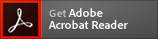Nisin from Lactococcus lactis An antibiotic with bactericidal action

# Nisin from Lactococcus lactis (CAS 1414-45-5)

Nisin from iLactococcus lactis/i | CAS 1414-45-5 is rated 5.0 out of 5 by 1.
Application: An antibiotic with bactericidal action
CAS Number: 1414-45-5
Molecular Weight: 3354.07
Molecular Formula: C143H230N42O37S7
* Refer to Certificate of Analysis for lot specific data (including water content).Antibiotic with bactericidal action. Binds to the lipid A portion of bacterial lipopolysaccharides. Induces pore formation in the membranes of cortex cells from excised sorghum roots. Mode of Action: Binds to and interferes with the permeability of the cytoplasmic membrane. Antimicrobial spectrum: Gram-negative and Gram-positive bacteria (requires chelator such as EDTA).

#### References

Cornu, J. Ann. Microbiol. 131B, 121, (1980) Storm, D.R., et al. Annu. Rev. Biochem. 46, 723, (1977) Morrison, D.C. and Jacobs, D.M. Immunochemistry 13, 813, (1976) Lerner, H.R., et al. Physiol. Plant. 57, 90, (1983)

Formulation :
2.5% (balance sodium chloride and denatured milk solids)
Appearance :
Powder
Physical State :
Solid
Storage :
Store at 4° C
For Research Use Only. Not Intended for Diagnostic or Therapeutic Use.
PubChem CID :
16133879
MDL Number :
MFCD00131724
SMILES :
CCC(C)C1C(=O)NC(=C)C(=O)NC(C(=O)NC(CSCC(C(=O)N1)NC(=O)/C(=C/C)/NC(=O)C(C(C)CC)N)C(=O)NC2C(SCC(NC(=O)CNC(=O)C3CCCN3C2=O)C(=O)NC(CCCCN)C(=O)NC4C(SCC(NC(=O)CNC(=O)C(NC(=O)C(NC(=O)C(NC(=O)CNC4=O)C)CC(C)C)CCSC)C(=O)NC(CC(=O)N)C(=O)NC(CCSC)C(=O)NC(CCCCN)C(=O)NC5C(SCC6C(=O)NC(C(=O)NC(CSC(C(C(=O)N6)NC(=O)C(NC5=O)C)C)C(=O)NC(CO)C(=O)NC(C(C)CC)C(=O)NC(CC7=CN=CN7)C(=O)NC(C(C)C)C(=O)NC(=C)C(=O)NC(CCCCN)C(=O)O)CC8=CN=CN8)C)C)C)CC(C)C

#### Certificate of AnalysisAdobe Acrobat Reader is required to reliably view,
print and comment on PDF documents